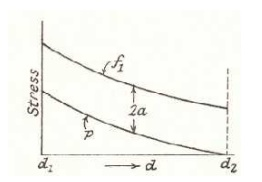Home | | Mechanics of Solids | Stresses on inclined plane

# Stresses on inclined planeprocedure to tackle stresses on inclined planes

Stresses on inclined plane

Stresses on inclined plane

procedure to tackle stresses on inclined planes

STEPS:

In order to do achieve the desired objective we proceed in the following manner

(i)       Label the Block ABCD.

(ii)     Set up axes for the direct stress (as abscissa) and shear stress (as ordinate)

(iii)   Plot the stresses on two adjacent faces e.g. AB and BC, using the following sign convention. Direct stresses tensile positive; compressive, negative

Shear stresses - tending to turn block clockwise, positive

- tending to turn block counter clockwise, negative

[ i.e shearing stresses are +ve when its movement about the centre of the element is clockwise ]

This gives two points on the graph which may than be labeled asrespectively to denote stresses these planes.

(iv)  Join   AB and BC(v)     The point P where this line cuts the s axis is than the centre of Mohr's stress circle and the li joining  AB and BCis diameter. Therefore the circle can now be drawn.

Now every point on the circle then represents a state of stress on some plane through C.

Study Material, Lecturing Notes, Assignment, Reference, Wiki description explanation, brief detail

Related Topics